# Texas Go Math Grade 7 Module 8 Answer Key Equations and Inequalities

Refer to our Texas Go Math Grade 7 Answer Key Pdf to score good marks in the exams. Test yourself by practicing the problems from Texas Go Math Grade 7 Module 8 Answer Key Equations and Inequalities.

## Texas Go Math Grade 7 Module 8 Answer Key Equations and Inequalities

Essential Question
How can you use equations and Inequalities to solve real-world problems?Complete these exercises to review skills you will need for this chapter.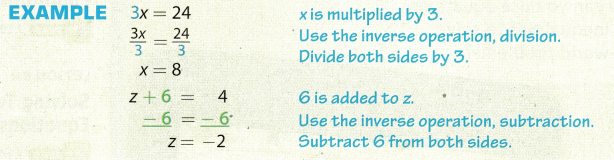Solve each equation, using inverse operations.

Question 1.
9w = -54 _______
Divide both sides by 9.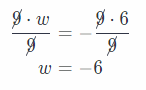Question 2.
b – 12 = 3 _______
Add 12 to both sides b – 12 + 12 = 3 + 12
b = 15

Question 3.
$$\frac{n}{4}$$ = -11 ____
Multiply both sides by 4.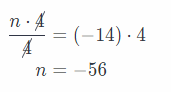Graph each number on the number line.Question 4.
33

Question 5.
-9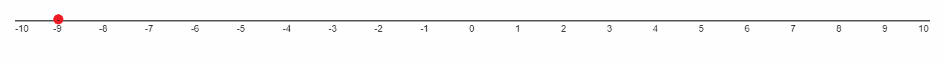-9

Question 6.
7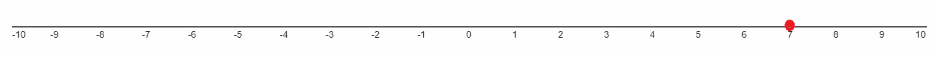7

Question 7.
-3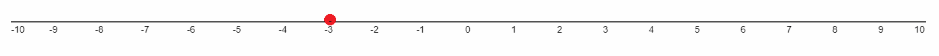-3

Question 8.
4p > 20 ______
Divide both sides by 4.Question 9.
m – 7 ≤ 3 ______
m + 7 + 7 ≤ 3 + 7
m ≤ 3 + 7
m ≤ 10

Question 10.
$$\frac{S}{-2}$$ < 9
Multiply both sides by -2. We are multiplying by a negative number, so we have to reverse direction of the inequality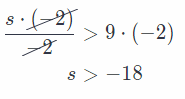Question 11.
r + 6 ≤ -7 ____
subtract 6 from both sides.
r + 6 – 6 ≤ -7 – 6
r ≤ -13

Question 12.
$$\frac{h}{4}$$ > -5 _____
Multiply both sides by 4.Question 13.
-y ≤ 2 ________
Multiply both sides by -1 We are multiplying by a negative number, so we have to reverse direction of the inequality.
-y . (-1) ≤ 2 . (-1)
y ≤ -2

Visualize Vocabulary

Use the ✓ words to complete the graphic. You may put more than one word in each box.Understand Vocabulary

Complete each sentence, using the review words.

Question 1.
A value of the variable that makes the equation true is a ____